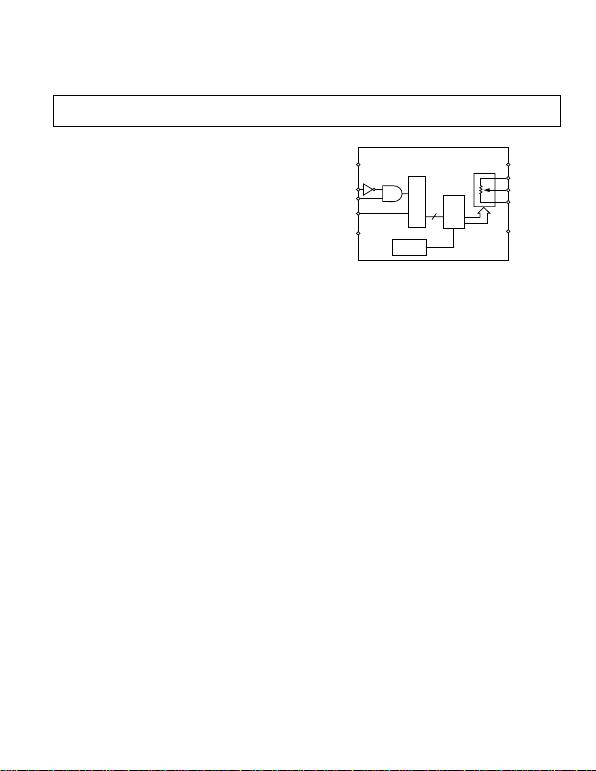Information furnished by Analog Devices is believed to be accurate and
reliable. However, no responsibility is assumed by Analog Devices for its
use, nor for any infringements of patents or other rights of third parties that
may result from its use. No license is granted by implication or otherwise
under any patent or patent rights of Analog Devices.
a
One Technology Way, P.O. Box 9106, Norwood, MA 02062-9106, U.S.A.
Tel: 781/329-4700 www.analog.com
FUNCTIONAL BLOCK DIAGRAM
SER
REG
PWR-ON
PRESET
A
W
B
RDAC
REG
Dx
8/6
CS
CLK
SDI
V
SS
V
DD
GND SHDN
256-Position and 33-Position
Digital Potentiometers
FEATURES
10 k, 50 k
3-Wire SPI-Compatible Serial Data Input
Single Supply 2.7 V to 5.5 V or
Dual Supply 2.7 V for AC or Bipolar Operations
Internal Power-On Midscale Preset
APPLICATIONS
Mechanical Potentiometer Replacement
Programmable Voltage-to-Current Conversion
Programmable Filters, Delays, Time Constants
Line Impedance Matching
GENERAL DESCRIPTION
with 256 positions and 33 positions respectively, that can be digi-
tally controlled through a 3-wire SPI serial interface. The terms
programmable resistor, variable resistor (VR), and RDAC are
commonly used interchangeably to refer to digital potentiometers.
These devices perform the same electronic adjustment function
contain a single variable resistor in the compact
package. Each device contains a fixed wiper resistance at the
wiper contact that taps the programmable resistance at a point
determined by a digital code. The code is loaded in the serial
input register. The resistance between the wiper and either end
point of the programmable resistor varies linearly with respect to
the digital code transferred into the VR latch. Each variable
resistor offers a completely programmable value of resistance,
between the A terminal and the wiper, or the B terminal and the
wiper. The fixed A-to-B terminal resistance of 10 k or 50 k
has a nominal temperature coefficient of 500 ppm/°C. The VR
has a VR latch that holds its programmed resistance value. The
VR latch is updated from an SPI-compatible serial-to-parallel
shift register that is loaded from a standard 3-wire serial-input
digital interface. Eight data bits for the AD5200 and six data
bits for the AD5201 make up the data word that is clocked into
the serial input register. The internal preset forces the wiper to
H
and 10
H
AD5201 VR latches respectively. The SHDN pin forces the
resistor to an end-to-end open-circuit condition on the A terminal
and shorts the wiper to the B terminal, achieving a microwatt
power shutdown state. When SHDN is returned to logic high,
the previous latch setting puts the wiper in the same resistance
setting prior to shutdown. The digital interface is still active dur-
ing shutdown so that code changes can be made that will produce
a new wiper position when the device is returned from shutdown.
All parts are guaranteed to operate over the extended industrial
temperature range of –40°C to +85°C.
REV. C
2012
781/461-3113
MSOP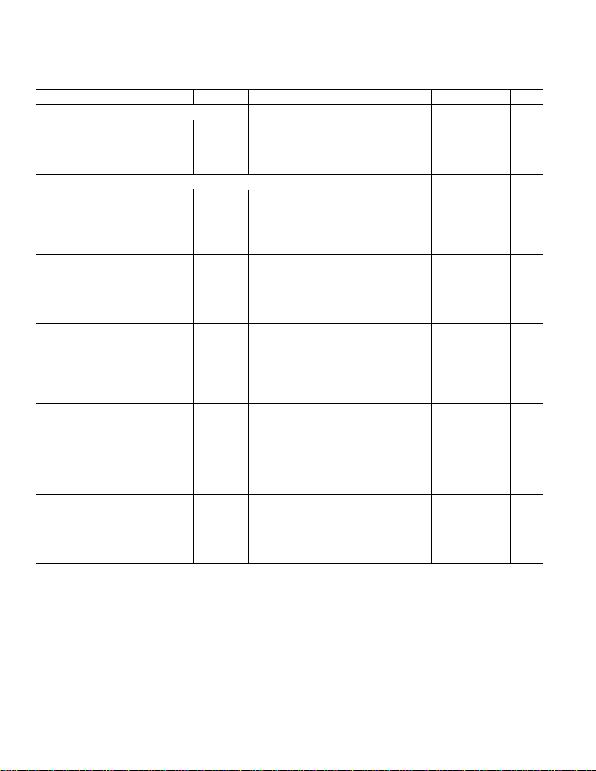–2–
Parameter Symbol Conditions Min Typ1Max Unit
DC CHARACTERISTICS RHEOSTAT MODE
Resistor Differential Nonlinearity 2R-DNL RWB, VA = No Connect –1 ±0.25 +1 LSB
Resistor Integral Nonlinearity 2R-INL RWB, VA = No Connect –2 ±0.5 +2 LSB
Nominal Resistor Tolerance 3RAB TA = 25°C –30 +30 %
Resistance Temperature Coefficient RAB/TV
AB = VDD, Wiper = No Connect 500 ppm/ °C
Wiper Resistance RWVDD = 5 V 50 100
DC CHARACTERISTICS POTENTIOMETER DIVIDER MODE (Specifications apply to all VRs.)
Resolution N 8 Bits
Differential Nonlinearity 4DNL 1 ±1/4 +1 LSB
Integral Nonlinearity 4INL 2 ±1/2 +2 LSB
Voltage Divider Temperature Coefficient VW/T Code = 80 H5 ppm/ °C
Full-Scale Error VWFSE Code = FF H–1.5 –0.5 0 LSB
Zero-Scale Error VWZSE Code = 00 H0 +0.5 +1.5 LSB
RESISTOR TERMINALS
Voltage Range 5VA, B, WVSS VDD V
Capacitance 6 A, B CA, Bf = 1 MHz, Measured to GND, Code = 80 H45 pF
Capacitance 6 WC
Wf = 1 MHz, Measured to GND, Code = 80 H60 pF
Shutdown Supply Current 7IDD_SD VDD = 5.5 V 0.01 5 µA
Common-Mode Leakage ICM VA = VB = VDD/2 1 nA
DIGITAL INPUTS AND OUTPUTS
Input Logic High VIH 2.4 V
Input Logic Low VIL 0.8 V
Input Logic High VIH VDD = 3 V, VSS = 0 V 2.1 V
Input Logic Low VIL VDD = 3 V, VSS = 0 V 0.6 V
Input Current IIL VIN = 0 V or 5 V ±1µA
Input Capacitance 6CIL 5pF
POWER SUPPLIES
Logic Supply VLOGIC 2.7 5.5 V
Power Single-Supply Range VDD RANGE VSS = 0 V –0.3 5.5 V
Power Dual-Supply Range VDD/SS RANGE ±2.3 ±2.7 V
Positive Supply Current IDD VIH = +5 V or VIL = 0 V 15 40 µA
Negative Supply Current ISS VSS = –5 V 15 40 µA
Power Dissipation 8PDISS VIH = +5 V or VIL = 0 V, VDD = +5 V, VSS = 0 V 0.2 mW
Power Supply Sensitivity PSS VDD = +5 V ± 10%, Code = Midscale –0.01 0.001 +0.01 %/%
DYNAMIC CHARACTERISTICS 6, 9
Bandwidth –3 dB BW_10 kRAB = 10 k, Code = 80 H600 kHz
BW_50 kRAB = 50 k, Code = 80 H100 kHz
Total Harmonic Distortion THDWVA = 1 V rms, VB = 0 V, f = 1 kHz, R AB = 10 k0.003 %
VW Settling Time (10 k/50 k)t
SVA = 5 V, VB = 0 V, ± 1 LSB Error Band 2/9 µs
Resistor Noise Voltage Density eN_WB RWB = 5 k, RS = 0 9 nVHz
NOTES
1
Typicals represent average readings at 25°C and V
DD
= 5 V, V
SS
= 0 V.
2
Resistor position nonlinearity error R-INL is the deviation from an ideal value measured between the maximum resistance and the minimum resistance wiper posi-
tions. R-DNL measures the relative step change from ideal between successive tap positions. Parts are guaranteed monotonic. I
W
= V
DD
/R for both V
DD
= +2.7 V,
V
SS
= –2.7 V.
3
V
AB
= V
DD
, Wiper (V
W
) = No connect.
4
INL and DNL are measured at V
W
with the RDAC configured as a potentiometer divider similar to a voltage output D/A converter. V
A
= V
DD
and V
B
= 0 V. DNL
specification limits of ±1 LSB maximum are Guaranteed Monotonic operating conditions.
5
Resistor Terminals A, B, W have no limitations on polarity with respect to each other.
6
Guaranteed by design and not subject to production test.
7
Measured at the A terminal. A terminal is open-circuited in shutdown mode.
8
P
DISS
is calculated from (I
DD
× V
DD
). CMOS logic level inputs result in minimum power dissipation.
9
All dynamic characteristics use V
DD
= 5 V, V
SS
= 0 V.
Specifications subject to change without notice.
(VDD = 5 V 10%, or 3 V 10%, VSS = 0 V, VA = +VDD, VB = 0 V,
–40C < TA < +85C unless otherwise noted.)
REV. C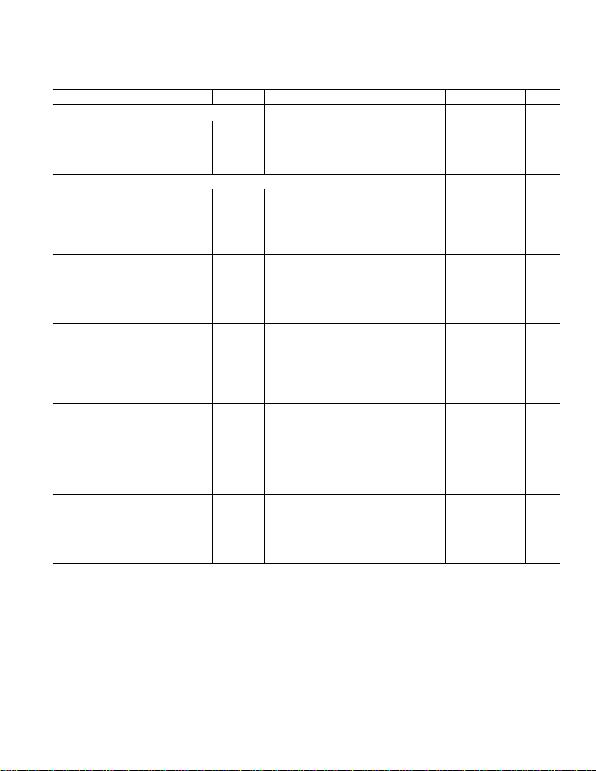–3–
(VDD = 5 V 10%, or 3 V 10%, VSS = 0 V, VA = +VDD, VB = 0 V,
–40C < TA < +85C unless otherwise noted.)
Parameter Symbol Conditions Min Typ1Max Unit
DC CHARACTERISTICS RHEOSTAT MODE
Resistor Differential Nonlinearity 2R-DNL RWB, VA = No Connect –0.5 ±0.05 +0.5 LSB
Resistor Integral Nonlinearity 2R-INL RWB, VA = No Connect –1 ±0.1 +1 LSB
Nominal Resistor Tolerance 3RAB TA = 25°C –30 +30 %
Resistance Temperature Coefficient RAB/TV
AB = VDD, Wiper = No Connect 500 ppm/ °C
Wiper Resistance RWVDD = 5 V 50 100
DC CHARACTERISTICS POTENTIOMETER DIVIDER MODE (Specifications apply to all VRs.)
Resolution 4N 6 Bits
Differential Nonlinearity 5DNL –0.5 ±0.01 +0.5 LSB
Integral Nonlinearity 5INL 1 ±0.02 +1 LSB
Voltage Divider Temperature Coefficient VW/T Code = 10 H5 ppm/ °C
Full-Scale Error VWFSE Code = 20 H–1/2 –1/4 0 LSB
Zero-Scale Error VWZSE Code = 00 H0 +1/4 +1/2 LSB
RESISTOR TERMINALS
Voltage Range 6VA, B, WVSS VDD V
Capacitance 7 A, B CA, Bf = 1 MHz, Measured to GND, Code = 10 H45 pF
Capacitance 7 WC
Wf = 1 MHz, Measured to GND, Code = 10 H60 pF
Shutdown Supply Current 8IDD_SD VDD = 5.5 V 0.01 5 µA
Common-Mode Leakage ICM VA = VB = VDD/2 1 nA
DIGITAL INPUTS AND OUTPUTS
Input Logic High VIH 2.4 V
Input Logic Low VIL 0.8 V
Input Logic High VIH VDD = 3 V, VSS = 0 V 2.1 V
Input Logic Low VIL VDD = 3 V, VSS = 0 V 0.6 V
Input Current IIL VIN = 0 V or 5 V ±1µA
Input Capacitance 7CIL 5pF
POWER SUPPLIES
Logic Supply VLOGIC 2.7 5.5 V
Power Single-Supply Range VDD RANGE VSS = 0 V –0.3 5.5 V
Power Dual-Supply Range VDD/SS RANGE ±2.3 ±2.7 V
Positive Supply Current IDD VIH = +5 V or VIL = 0 V 15 40 µA
Negative Supply Current ISS VSS = –5 V 15 40 µA
Power Dissipation 9PDISS VIH = +5 V or VIL = 0 V, VDD = +5 V, VSS = –5 V 0.2 mW
Power Supply Sensitivity PSS VDD = +5 V ± 10% –0.01 0.001 +0.01 %/%
DYNAMIC CHARACTERISTICS 7, 10
Bandwidth –3 dB BW_10 kRAB = 10 k, Code = 10 H600 kHz
BW_50 kRAB = 50 k, Code = 10 H100 kHz
Total Harmonic Distortion THDWVA = 1 V rms, VB = 0 V, f = 1 kHz, R AB = 10 k0.003 %
VW Settling Time (10 k/50 k)t
SVA = 5 V, VB = 0 V, ± 1 LSB Error Band 2/9 µs
Resistor Noise Voltage Density eN_WB RWB = 5 k, RS = 0 9 nVHz
NOTES
1
Typicals represent average readings at 25°C and V
DD
= 5 V, V
SS
= 0 V.
2
Resistor position nonlinearity error R-INL is the deviation from an ideal value measured between the maximum resistance and the minimum resistance wiper posi-
tions. R-DNL measures the relative step change from ideal between successive tap positions. Parts are guaranteed monotonic. I
W
= V
DD
/R for both V
DD
= +2.7 V,
V
SS
= –2.7 V.
3
V
AB
= V
DD
, Wiper (V
W
) = No connect.
4
Six bits are needed for 33 positions even though it is not a 64-position device.
5
INL and DNL are measured at V
W
with the RDAC configured as a potentiometer divider similar to a voltage output D/A converter. V
A
= V
DD
and V
B
= 0 V. DNL
specification limits of ±1 LSB maximum are Guaranteed Monotonic operating conditions.
6
Resistor Terminals A, B, W have no limitations on polarity with respect to each other.
7
Guaranteed by design and not subject to production test.
8
Measured at the A terminal. A terminal is open-circuited in shutdown mode.
9
P
DISS
is calculated from (I
DD
× V
DD
). CMOS logic level inputs result in minimum power dissipation.
10
All dynamic characteristics use V
DD
= 5 V, V
SS
= 0 V.
Specifications subject to change without notice.
REV. C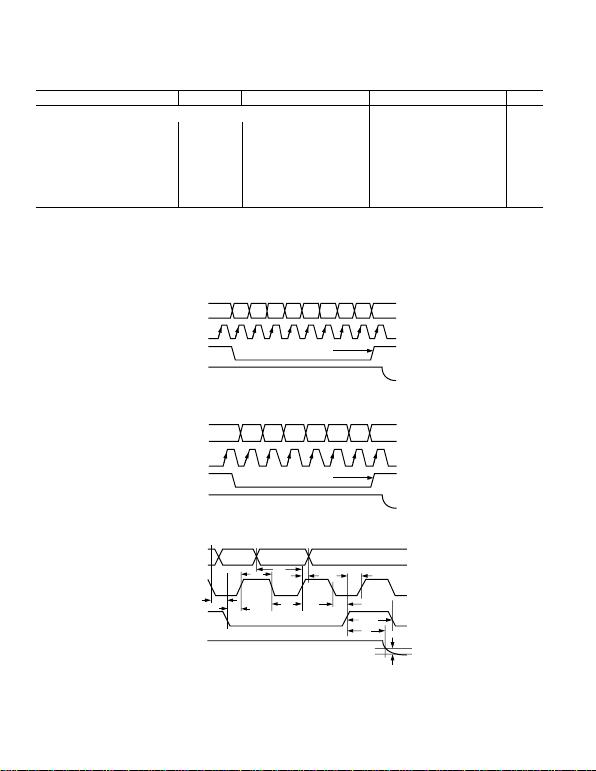–4–
ELECTRICAL CHARACTERISTICS
Parameter Symbol Conditions Min Typ
1
Max Unit
INTERFACE TIMING CHARACTERISTICS (Applies to All Parts [Notes 2, 3])
Input Clock Pulsewidth t
CH
, t
CL
Clock Level High or Low 20 ns
Data Setup Time t
DS
5ns
Data Hold Time t
DH
5ns
CS Setup Time t
CSS
15 ns
CS High Pulsewidth t
CSW
40 ns
CLK Fall to CS Fall Hold Time t
CSH0
0ns
CLK Fall to CS Rise Hold Time t
CSH1
0ns
CS Rise to Clock Rise Setup t
CS1
10 ns
NOTES
1
Typicals represent average readings at 25°C and V
DD
= 5 V, V
SS
= 0 V.
2
Guaranteed by design and not subject to production test.
3
See timing diagram for location of measured values. All input control voltages are specified with t
R
= t
F
= 2 ns (10% to 90% of 3 V) and timed from a voltage level of
1.5 V. Switching characteristics are measured using V
LOGIC
= 5 V.
Specifications subject to change without notice.
(VDD = 5 V 10%, or 3 V 10%, VSS = 0 V, VA = +VDD, VB = 0 V, –40C < TA < +85C
unless otherwise noted.)
D7 D6 D5 D4 D3 D2 D1 D0
0
1
SDI
0
1
CLK
0
1
VOUT
0
1
0
1
SDI D5D4D3D2D1D0
0
1
CLK
0
1
0
1
VOUT
Dx Dx
0
1
0
1
0
1
0
V
DD
SDI
(DATA IN)
CLK
CS
VOUT
tCH
tDS tDH tCS1
tCSW
tS
tCL
tCSH0
tCSS
1LSB
tCSH1
Figure 1c. Detail Timing Diagram
REV. C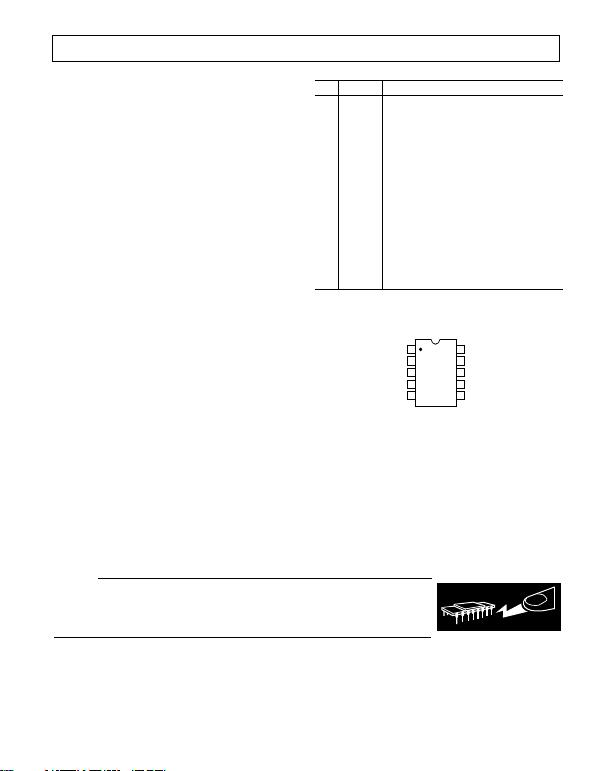–5–
ABSOLUTE MAXIMUM RATINGS
1
(T
A
= 25°C, unless otherwise noted)
V
DD
to V
SS
. . . . . . . . . . . . . . . . . . . . . . . . . . . . . . . . . . . . . 7 V
V
DD
to GND . . . . . . . . . . . . . . . . . . . . . . . . . . . . . 0.3, +7 V
V
SS
to GND . . . . . . . . . . . . . . . . . . . . . . . . . . . . . . . 0 V, 7 V
V
A
, V
B
, V
W
to GND . . . . . . . . . . . . . . . . . . . . . . . . . V
SS
, V
DD
I
MAX
. . . . . . . . . . . . . . . . . . . . . . . . . . . . . . . . . . . . . ±20 mA
2
Digital Inputs and Output Voltage to GND . . . . . . . 0 V, 7 V
Operating Temperature Range . . . . . . . . . . . 40°C to +85°C
Maximum Junction Temperature (T
J
Max) . . . . . . . . . 150°C
Storage Temperature . . . . . . . . . . . . . . . . . . 65°C to +150°C
Lead Temperature (Soldering, 10 sec) . . . . . . . . . . . . 300°C
Thermal Resistance θ
JA,
. . . . . . . . . . . . . 200°C/W
Package Power Dissipation = (T
J
Max T
A
)/θ
JA
NOTES
1
Stresses above those listed under Absolute Maximum Ratings may cause perma-
nent damage to the device. This is a stress rating; functional operation of the device
at these or any other conditions above those listed in the operational sections of this
specification is not implied. Exposure to absolute maximum rating conditions for
extended periods may affect device reliability.
2
Max current is bounded by the maximum current handling of the switches,
maximum power dissipation of the package, and maximum applied voltage across
any two of the A, B, and W terminals at a given resistance. Please refer to TPC 31
and TPC 32 for detail.
PIN CONFIGURATION
TOP VIEW
(Not to Scale)
10
9
8
7
6
1
2
3
4
5
B
VSS
GND
CS
SDI
A
W
VDD
SHDN
CLK
CAUTION
ESD (electrostatic discharge) sensitive device. Electrostatic charges as high as 4000 V readily
accumulate on the human body and test equipment and can discharge without detection. Although
on devices subjected to high-energy electrostatic discharges. Therefore, proper ESD precautions
are recommended to avoid performance degradation or loss of functionality.
WARNING!
ESD SENSITIVE DEVICE
PIN FUNCTION DESCRIPTIONS
Pin Name Description
1 B B Terminal.
2V
SS
Negative Power Supply, specified for opera-
tion from 0 V to 2.7 V.
3 GND Ground.
4CS Chip Select Input, Active Low. When CS
returns high, data will be loaded into the
DAC register.
5 SDI Serial Data Input.
6 CLK Serial Clock Input, positive edge triggered.
7SHDN Active Low Input. Terminal A open circuit.
Shutdown controls Variable Resistors of
RDAC to temporary infinite.
8V
DD
Positive Power Supply (Sum of V
DD
+ V
SS
5.5 V).
9 W Wiper Terminal.
10 A A Terminal.
REV. C
MSOP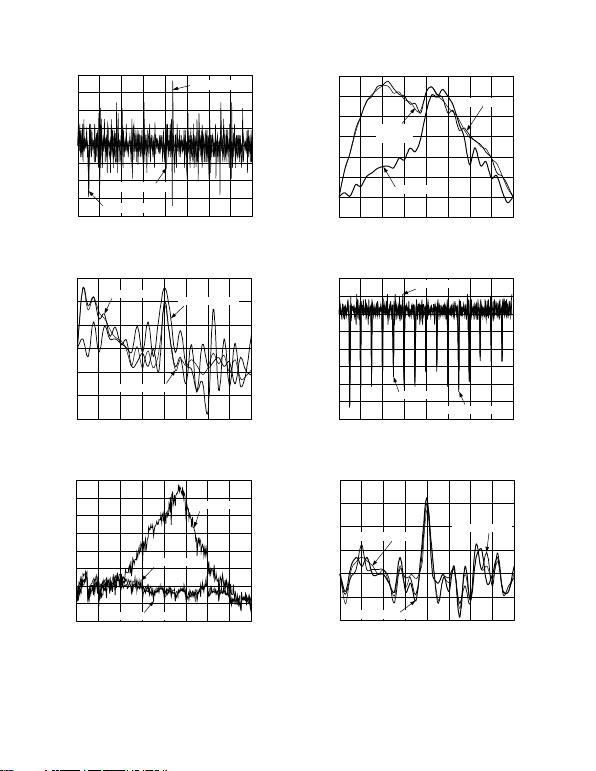–6–
CODE – Decimal
0.20
RDNL – LSB
224
0.15
0.10
0.05
0.00
0.05
0.10
0.15
0.20
1921601289664320 256
V
DD
= 2.7V, V
SS
= 0V
V
DD
= 5.5V, V
SS
= 0V
V
DD
= +2.7V, V
SS
= –2.7V
RDNL vs. Code
CODE Decimal
RDNL LSB
28
0.02
0.01
0.00
0.01
0.02
0.03
2420161284032
0.03
V
DD
= 2.7V, V
SS
= 0V
V
DD
= 5.5V, V
SS
= 0V
V
DD
= +2.7V, V
SS
= 2.7V
RDNL vs. Code
CODE Decimal
RINL LSB
224
0.0
0.1
0.2
0.3
0.5
0.7
1921601289664320 256
0.1
V
DD
= 2.7V, V
SS
= 0V
V
DD
= 5.5V, V
SS
= 0V
V
DD
= +2.7V, V
SS
= 2.7V
0.6
0.4
RINL vs. Code
CODE Decimal
RINL LSB
28
0.00
0.02
0.04
0.06
0.08
0.12
2420161284032
0.02
V
DD
= +2.7V
V
SS
= 2.7V
V
DD
= 2.7V, V
SS
= 0V
V
DD
= 5.5V, V
SS
= 0V
0.10
RINL vs. Code
CODE Decimal
DNL LSB
224
0.25
0.20
0.15
0.10
0.05
0.10
1921601289664320 256
0.30
V
DD
= +2.7V, V
SS
= 2.7V
V
DD
= 5.5V, V
SS
= 0V
V
DD
= 2.7V, V
SS
= 0V
0.00
0.05
DNL vs. Code
CODE Decimal
DNL LSB
28
0.005
0.000
0.005
0.010
0.020
2420161284032
0.010
VDD = +2.7V, VSS = 2.7V
VDD = 5.5V, VSS = 0V
VDD = 2.7V, VSS = 0V
0.015
DNL vs. Code
REV. C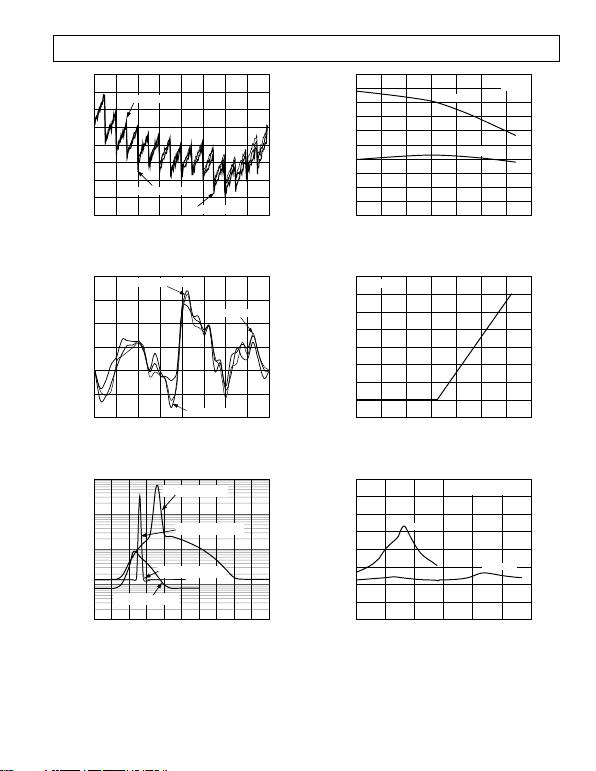–7–
CODE Decimal
INL LSB
224
0.2
0.1
0.0
0.1
0.3
1921601289664320 256
0.3
V
DD
= +2.7V, V
SS
= 2.7V
V
DD
= 5.5V, V
SS
= 0V
V
DD
= 2.7V, V
SS
= 0V
0.2
0.4
0.5
INL vs. Code
CODE Decimal
INL LSB
28
0.005
0.010
0.020
2420161284032
0.000
VDD = +2.7V, VSS = 2.7V
VDD = 5.5V, VSS = 0V
VDD = 2.7V, VSS = 0V
0.015
0.005
0.010
INL vs. Code
VIH V
IDD/ISS mA
0.01
0.1
10
5.04.03.02.01.00.0
0.001
1.0
IDD @ VDD/VSS = 5V/0V
IDD @ VDD/VSS = 3V/0V
IDD @ VDD/VSS = 2.5V
ISS @ VDD/VSS = 2.5V
TPC 9. Supply Current vs. Logic Input Voltage
TPC 10. Supply Current vs. Temperature
TPC 11. Shutdown Current vs. Temperature
V
SUPPLY
V
R
ON
160
06
140
120
100
80
60
40
20
0
54321
V
DD
= 2.7V
V
DD
= 5.5V
SEE TEST CIRCUIT 13
T
A
= 25C
TPC 12. Wiper ON Resistance vs. V
SUPPLY
REV. C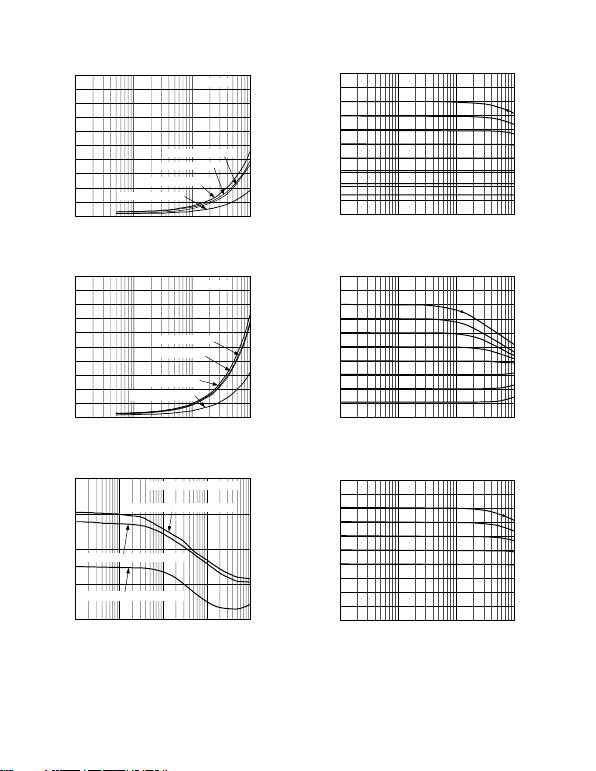–8–
FRE
Q
UENCY Hz
IDD/ISS A
500
10k
450
400
350
300
250
200
150
100
50
0
100k 1M 10M
CODE FFH
ISS @ VDD/VSS = 2.5V
IDD @ VDD/VSS = 2.5V
IDD @ VDD/VSS = 5V/0V
IDD @ VDD/VSS = 3V/0V
Supply Current vs. Clock Frequency
FREQUENCY Hz
I
DD
/I
SS
A
500
10k
450
400
350
300
250
200
150
100
50
0
100k 1M 10M
CODE 55
H
I
SS
@ V
DD
/V
SS
= 2.5V
I
DD
@ V
DD
/V
SS
= 2.5V
I
DD
@ V
DD
/V
SS
= 5V/0V
I
DD
@ V
DD
/V
SS
= 3V/0V
Supply Current vs. Clock Frequency
FREQUENCY Hz
PSRR
dB
100 1k 10k 1M
+PSRR
@ V
DD
= 5V DC 10% p-p AC
100k
+PSRR
@ V
DD
= 3V DC 10% p-p AC
PSRR
@ V
DD
= 3V DC 10% p-p AC
CODE = 80
H
, V
A
= V
DD
, V
B
= 0V
80
60
40
20
0
TPC 15. Power Supply Rejection Ratio vs. Frequency
FREQUENCY Hz
6
54
GAIN dB
1k 10k 100k 1M
48
42
36
30
24
18
12
6
0
80H
40H
20H
10H
08H
04H
02H
01H
Gain vs. Frequency vs. Code
FREQUENCY Hz
6
54
GAIN dB
1k 10k 100k 1M
48
42
36
30
24
18
12
6
0
80H
40H
20H
10H
08H
04H
02H
01H
Gain vs. Frequency vs. Code
FREQUENCY Hz
6
54
GAIN dB
1k 10k 100k 1M
48
42
36
30
24
18
12
6
0
10H
8H
4H
2H
1H
Gain vs. Frequency vs. Code
REV. C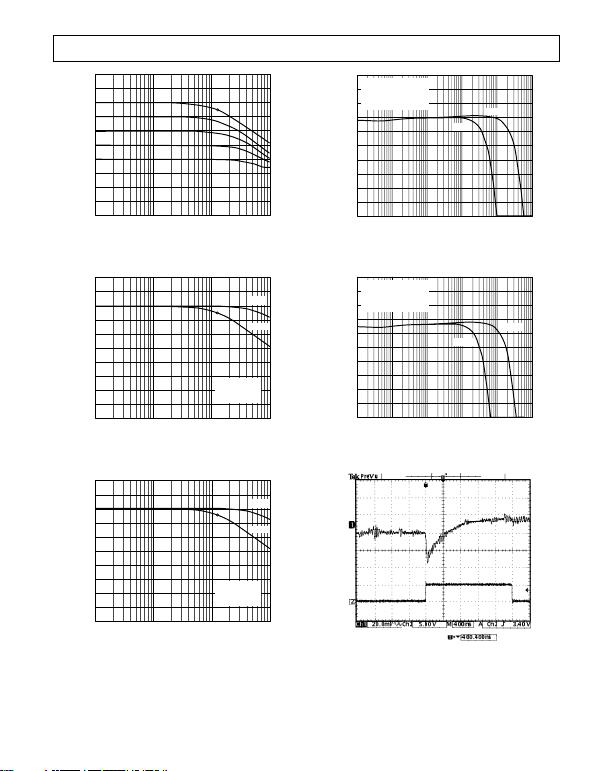–9–
FREQUENCY Hz
6
54
GAIN dB
1k 10k 100k 1M
48
42
36
30
24
18
12
6
0
10H
8H
4H
2H
1H
Gain vs. Frequency vs. Code
FREQUENCY Hz
12
48
GAIN dB
1k 10k 100k 1M
42
36
30
24
18
12
6
0
6
10k
VIN = 100mV rms
VDD = 5V
RL = 1M
50k
TPC 20. AD5200 –3 dB Bandwidth
FREQUENCY Hz
12
48
GAIN dB
1k 10k 100k 1M
42
36
30
24
18
12
6
0
6
10k
VIN = 100mV rms
VDD = 5V
RL = 1M
50k
TPC 21. AD5201 –3 dB Bandwidth
FREQUENCY Hz
12
48
NORMALIZED GAIN FLATNESS 0.1dB/DIV
10 10k 100k 1M
42
36
30
24
18
12
6
0
6
100 1k
50k
10k
SEE TEST CIRCUIT 10
CODE = 80H
VDD = 5V
TA = 25C
TPC 22. Normalized Gain Flatness vs. Frequency
TPC 23. AD5201 Normalized Gain Flatness vs. Frequency
VW
(20mV/DIV)
CS
(5V/DIV)
TPC 24. One Position Step Change at Half Scale
REV. C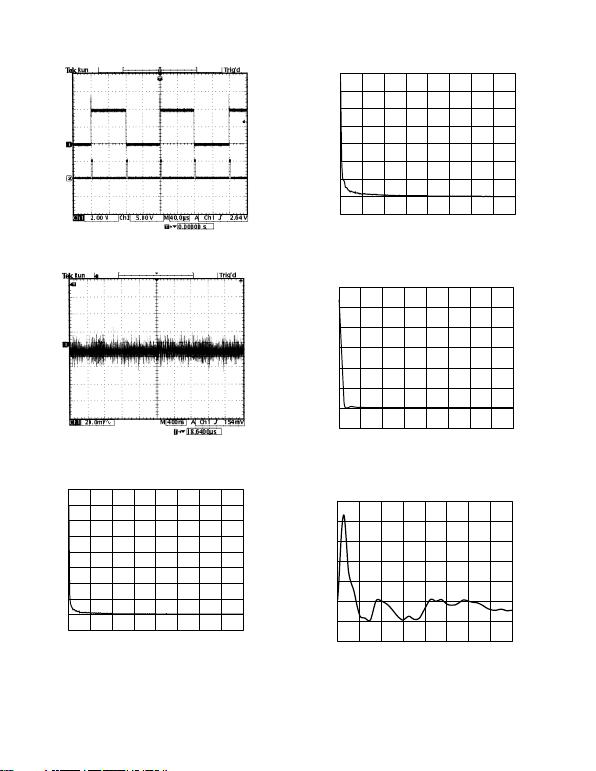–10–
OUTPUT
(2V/DIV)
INPUT
(5V/DIV)
TPC 25. Large Signal Settling Time
VOUT
(20mV/DIV)
TPC 26. Digital Feedthrough vs. Time
CODE Decimal
4000
500
POTENTIOMETER MODE TEMPCO ppm/C
0
3500
3000
2500
2000
1500
1000
500
0
32 64 96 128 160 192 224 256
V
WB
/
T Potentiometer Mode
Temperature Coefficient
CODE Decimal
500
RHEOSTAT MODE TEMPCO ppm/C
0
3500
3000
2500
2000
1500
1000
500
0
32 64 96 128 160 192 224 256
R
WB
/
T Rheostat Mode Temperature
Coefficient
CODE Decimal
POTENTIOMETER MODE TEMPCO ppm/C
3000
0
2500
2000
1500
1000
500
0
500
4 8 12 16 20 24 28 32
TPC 29. AD5201 Potentiometer Mode Temperature
Coefficient
CODE Decimal
POTENTIOMETER MODE TEMPCO ppm/C
50
0
40
30
20
10
0
20
4 8 12 16 20 24 28 32
1
0
V
WB
/
T Potentiometer Mode Tempco
REV. C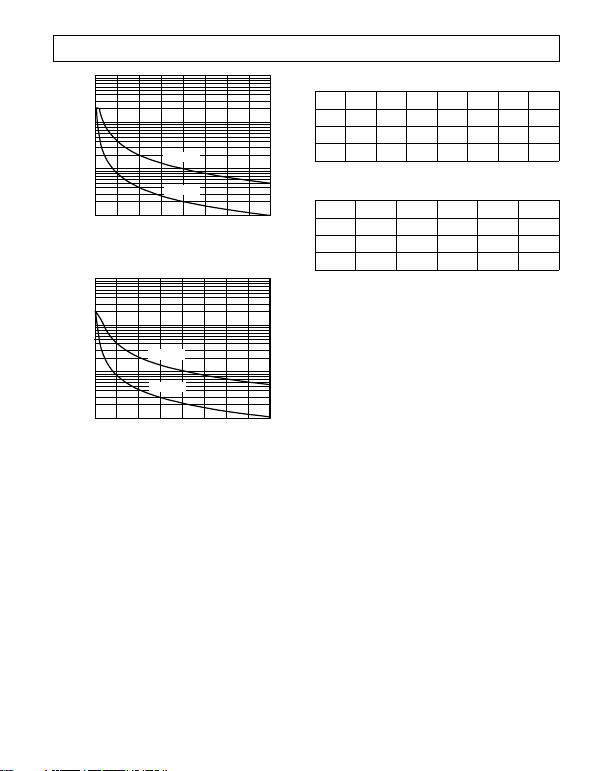–11–
CODE Decimal
100.0
10.0
0.1032
THEORETICAL I
MAX
mA
1.0
64 96 128 160 192 224 256
R
AB
= 10k
R
AB
= 50k
MAX
vs. Code
CODE Decimal
100.0
10.0
0.104
THEORETICAL I
MAX
mA
1.0
812
16 20 24 28 32
R
AB
= 10k
R
AB
= 50k
MAX
vs. Code
OPERATION
controlled variable resistor (VR) devices. Changing the
programmed VR settings is accomplished by clocking in an 8-bit
serial data word for AD5200, and a 6-bit serial data word for
AD5201, into the SDI (Serial Data Input) pins. Table I provides
preset to a midscale internally during power-on condition. In
SHDN pins that place the RDAC in a zero power consump-
tion state where the immediate switches next to Terminals A and
B are open-circuited. Meanwhile, the wiper W is connected to B
terminal, resulting in only leakage current consumption in the VR
structure. During shutdown, the VR latch contents are maintained
when the RDAC is inactive. When the part is returned from
shutdown, the stored VR setting will be applied to the RDAC.
Table I. AD5200 Serial-Data Word Format
7B6B5B4B3B2B1B0B
7D6D5D4D3D2D1D0D
BSMBSL
2
7
2
0
Table II. AD5201 Serial-Data Word Format
5B *4B3B2B1B0B
5D *4D3D2D1D0D
BSMBSL
2
5
2
0
*Six data bits are needed for 33 positions.
PROGRAMMING THE VARIABLE RESISTOR
Rheostat Operation
The nominal resistance of the RDAC between Terminals A and
B are available with values of 10 k and 50 k. The final two
digits of the part number determine the nominal resistance
value, e.g., 10 k = 10 and 50 k = 50. The nominal resistance
(R
AB
) of AD5200 has 256 contact points accessed by the wiper
terminal. The 8-bit data word in the RDAC latch of AD5200 is
decoded to select one of the 256 possible settings. In both parts,
the wipers first connection starts at the B terminal for data 00
H
.
This B-terminal connection has a wiper contact resistance of
50 as long as valid V
DD
/V
SS
is applied, regardless of the nominal
resistance. For a 10 k part, the second connection of AD5200 is
the first tap point with 89 [R
WB
= R
AB
/255 + R
W
= 39 + 50 ]
for data 01
H
. The third connection is the next tap point representing
78 + 50 = 128 for data 02
H
. Due to its unique internal structure,
AD5201 has 5-bit + 1 resolution, but needs a 6-bit data word to
achieve the full 33 steps resolution. The 6-bit data word in the
RDAC latch is decoded to select one of the 33 possible settings.
Data 34 to 63 will automatically be equal to Position 33. The
wiper 00
H
connection of AD5201 gives 50 . Similarly, for a
10 k part, the first tap point of AD5201 yields 363 for
data 01
H
, 675 for data 02
H
each LSB data value increase moves the wiper up the resistor
ladder until the last tap point is reached. Figures 2a and 2b show
the simplified diagrams of the equivalent RDAC circuits.
REV. C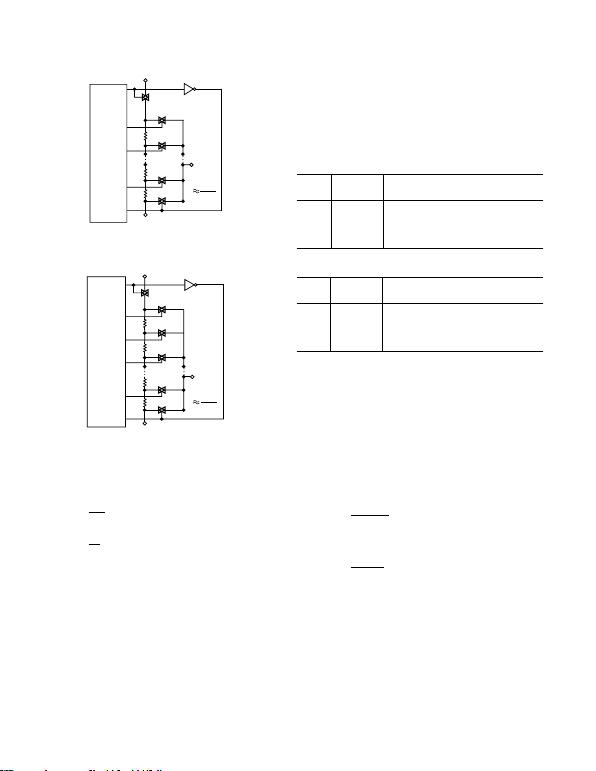–12–
D7
D6
D5
D4
D3
D2
D1
D0
RDAC
LATCH &
DECODER
SHDN
SW
SHDN
SW
2N1
SW
2N2
SW
1
SW
0
A
R
R
R
B
W
DIGITAL CIRCUITRY
OMITTED FOR CLARITY
RR
AB
2
N
1
Figure 2a. AD5200 Equivalent RDAC Circuit. 255 positions
can be achieved up to Switch SW
2
N–1
.
SW
2N1
D5
D4
D3
D2
D1
D0
RDAC
LATCH &
DECODER
SW
2N2
SW
1
SW
0
R
R
R
R
B
W
DIGITAL CIRCUITRY
OMITTED FOR CLARITY
SHDN
A
SW
SHDN
SW
2N
RR
AB
2
N
33 positions can be achieved all the way to Switch SW
2
N
.
The general equation determining the digitally programmed
output resistance between W and B is:
RD DR
WB AB
()
=+
255 50
RD DR
WB AB
()
=+
32 50
where:
Dis the decimal equivalent of the data contained in
RDAC latch.
R
AB
is the nominal end-to-end resistance.
R
W
is the wiper resistance contributed by the on-resistance
of the internal switch.
Note D in AD5200 is between 0 to 255 for 256 positions. On
the other hand, D in AD5201 is between 0 to 32 so that 33
positions can be achieved due to the slight internal structure
difference, Figure 2b.
Again if R
AB
= 10 k and A terminal can be opened or tied to
W, the following output resistance between W to B will be set
for the following RDAC latch codes:
DR
WB
(DEC) () Output State
255 10050 Full-Scale (R
AB
+ R
W
)
128 5070 Midscale
189 1 LSB
0 50 Zero-Scale (Wiper Contact Resistance)
DR
WB
(DEC) () Output State
32 10050 Full-Scale (R
AB
+ R
W
)
16 5050 Midscale
1 363 1 LSB
0 50 Zero-Scale (Wiper Contact Resistance)
Note that in the zero-scale condition a finite wiper resistance of
50 is present. Care should be taken to limit the current flow
between W and B in this state to no more than ±20 mA to avoid
degradation or possible destruction of the internal switch contact.
Like the mechanical potentiometer the RDAC replaces, it is
totally symmetrical. The resistance between the wiper W and
Terminal A also produces a digitally controlled resistance R
WA
.
When these terminals are used, the B terminal should be tied to
the wiper. Setting the resistance value for R
WA
starts at a maxi-
mum value of resistance and decreases as the data loaded in
the latch is increased in value. The general equation for this
operation is:
RD
D
R
WA AB
()
=
()
+
255
255 50
RD
D
R
WA AB
()
=
()
+
32
32 50
Similarly, D in AD5200 is between 0 to 255, whereas D in
AD5201 is between 0 to 32.
For R
AB
= 10 k and B terminal is opened or tied to the wiper
W, the following output resistance between W and A will be set
for the following RDAC latch codes:
REV. C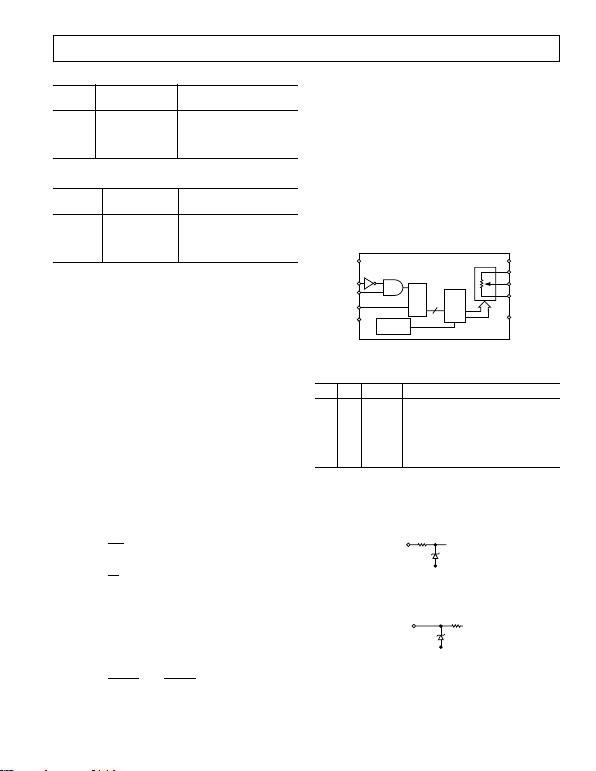–13–
DR
WA
(DEC) () Output State
255 50 Full-Scale (R
W
)
128 5030 Midscale
1 10011 1 LSB
0 10050 Zero-Scale (R
AB
+ R
W
)
DR
WA
(DEC) () Output State
32 50 Full-Scale (R
W
)
16 5050 Midscale
1 9738 1 LSB
0 10050 Zero-Scale (R
AB
+ R
W
)
The tolerance of the nominal resistance can be ±30% due to
process lot dependance. If users apply the RDAC in rheostat
(variable resistance) mode, they should be aware of such specifi-
cation of tolerance. The change in R
AB
with temperature has a
500 ppm/°C temperature coefficient.
PROGRAMMING THE POTENTIOMETER DIVIDER
Voltage Output Operation
The digital potentiometer easily generates output voltages at
wiper-to-B and wiper-to-A to be proportional to the input volt-
age at A to B.
Unlike the polarity of V
DD
V
SS
, which must be positive, volt-
age across AB, WA, and WB can be at either polarity.
If ignoring the effects of the wiper resistance for an approxima-
tion, connecting A terminal to 5 V and B terminal to ground
produces an output voltage at the wiper which can be any value
starting at almost zero to almost full scale with the minor devia-
tion contributed by the wiper resistance. Each LSB of voltage is
equal to the voltage applied across Terminal AB divided by the
2
N
-1
and 2
N
position resolution of the potentiometer divider for
ing the output voltage with respect to ground for any valid input
voltage applied to Terminals A and B is:
VD DVV
WABB
()
=+
255
VD DVV
WABB
()
=+
32
where D in AD5200 is between 0 to 255 and D in AD5201 is
between 0 to 32.
For more accurate calculation, including the effects of wiper
resistance, V
W
can be found as:
VD
RD
R
V
RD
R
V
W
WB
AB
A
WA
AB
B
()
=
()
+
()
(7)
where R
WB
(D) and R
WA
(D) can be obtained from Equations
1 to 4.
Operation of the digital potentiometer in the divider mode results
in more accurate operation over temperature. Here the output
voltage is dependent on the ratio of the internal resistors and not
the absolute values; therefore, the drift reduces to 15 ppm/°C.
DIGITAL INTERFACING
control interface. The three inputs are clock (CLK), CS, and
serial data input (SDI). The positive-edge-sensitive CLK input
requires clean transitions to avoid clocking incorrect data into
the serial input register. Standard logic families work well. If
mechanical switches are used for product evaluation, they
should be debounced by a flip-flop or other suitable means.
Figure 3 shows more detail of the internal digital circuitry. When
CS is low, the clock loads data into the serial register on each
positive clock edge (see Table III).
SER
REG
PWR-ON
PRESET
VSS
A
W
B
SHDN
RDAC
REG
Dx
8/6
VDD
CS
CLK
SDI
GND
Figure 3. Block Diagram
Table III. Input Logic Control Truth Table
CLK CS SHDN Register Activity
L L H No SR effect.
P L H Shift one bit in from the SDI pin.
X P H Load SR data into RDAC latch.
X H H No operation.
X H L Open circuit on A terminal and short
circuit between W to B terminals.
NOTE
P = positive edge, X = dont care, SR = shift register.
All digital inputs are protected with a series input resistor and
parallel Zener ESD structure shown in Figure 4. Applies to
digital input pins CS, SDI, SHDN, CLK.
340LOGIC
VSS
Figure 4. ESD Protection of Digital Pins
A,B,W
VSS
Figure 5. ESD Protection of Resistor Terminals
REV. C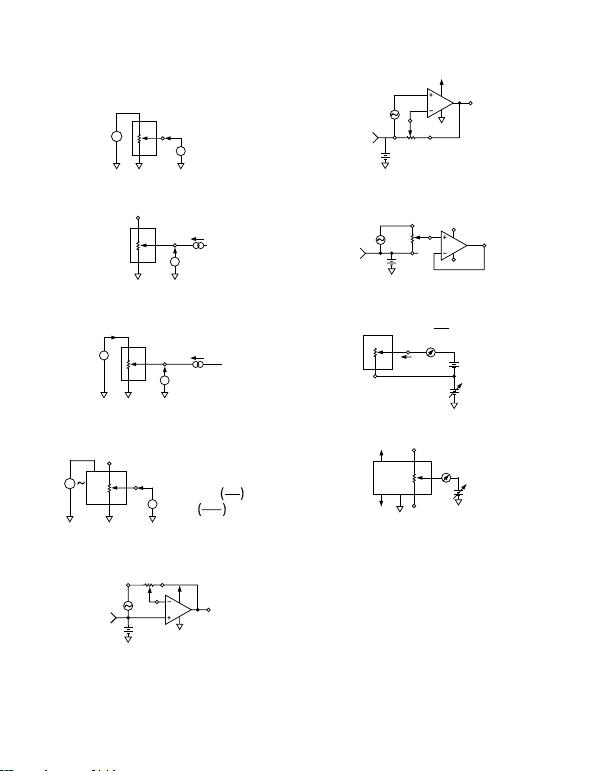–14–
TEST CIRCUITS
Figures 6 to 14 define the test conditions used in the product
specification table.
VMS
A
W
B
DUT
V+
V+ = VDD
1 LSB = V+/2N
Figure 6. Potentiometer Divider Nonlinearity Error Test
Circuit (INL, DNL)
VMS
A
W
B
DUT
NO CONNECT
IW
Figure 7. Resistor Position Nonlinearity Error
(Rheostat Operation; R-INL, R-DNL)
V
MS1
A
W
B
DUT
I
W
= V
DD
/R
NOMINAL
V
MS2
V
W
R
W
= [V
MS1
V
MS2
]/I
W
Figure 8. Wiper Resistance Test Circuit
V
MS
%
V
DD
%
PSS (%/%) =
V+ = V
DD
10%
PSRR (dB) = 20 LOG
V
MS
V
DD
V
MS
A
W
B
V+
V
DD
V
A
Figure 9. Power Supply Sensitivity Test Circuit
(PSS, PSRR)
OP279
W
5V
B
V
OUT
OFFSET
GND
OFFSET BIAS
A DUT
V
IN
Figure 10. Inverting Gain Test Circuit
OFFSET BIAS
B
OFFSET
GND A DUT
OP279
W
5V
VOUT
VIN
Figure 11. Noninverting Gain Test Circuit
OP42 VOUT
VIN
+15V
OFFSET
GND
15V
W
B
A
2.5V
Figure 12. Gain vs. Frequency Test Circuit
W
B
V
SS
TO V
DD
DUT
I
SW
CODE = OO
H
R
SW
=0.1V
I
SW
0.1V
+
Figure 13. Incremental ON Resistance Test Circuit
I
CM
A
W
B
NC
GND
NC
V
SS
V
DD
DUT
V
CM
NC = NO CONNECT
Figure 14. Common-Mode Leakage Current Test Circuit
REV. C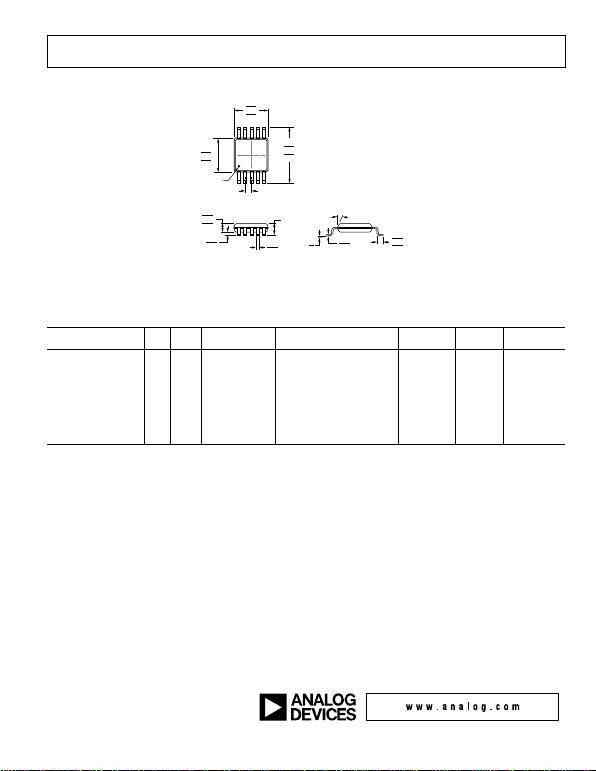REV. C –15–
OUTLINE DIMENSIONS
Figure 15. 10-Lead Mini Small Outline Package [MSOP]
(RM-10)
Dimensions shown in millimeters
ORDERING GUIDE
Model1 RES
Temperature
Range Package Description
Package
Option
Full Reel
Qty.
Branding
Information
1 Z = RoHS Compliant Part.
REVISION HISTORY
6/12—Rev. B to Rev. C
Removed Digital Potentiometer Selection Guide ....................... 15
Updated Outline Dimensions ........................................................ 15
Changes to Ordering Guide ........................................................... 15
8/01—Rev. A to Rev. B
Edits to ORDERING GUIDE .......................................................... 5
2/01—Rev. 0 to Rev. A
Edits to ORDERING GUIDE .......................................................... 5
Edits to ABSOLUTE MAXIMUM RATINGS ............................... 5
TPCs 31 and 32 added .................................................................... 11
COMPLIANT TO JEDEC STANDARDS MO-187-BA
091709-A
0.70
0.55
0.40
5
10
1
6
0.50 BSC
0.30
0.15
1.10 MAX
3.10
3.00
2.90
COPLANARITY
0.10
0.23
0.13
3.10
3.00
2.90
5.15
4.90
4.65
PIN 1
IDENTIFIER
15° MAX
0.95
0.85
0.75
0.15
0.05